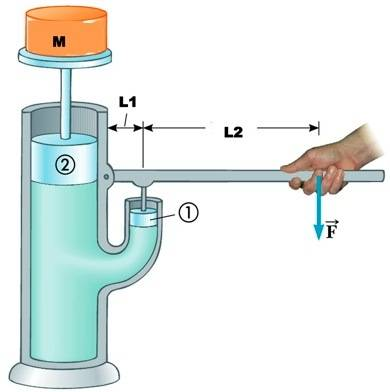# Pascal's principle hydraulic lift with torque involved

fordy314
Pascal's principle hydraulic lift with torque involved(need help with lever)

## Homework Statement

A hydraulic jack is used to support a mass which has a mass of 30.7 kg.The mass is supported by piston 2 which has a mass of 0.250 kg and an area of 40.2 cm2 . A force is applied by the lever on piston 1 which has a mass of 0.085 kg and an area of 14.2 cm2.

The distance between the hinge and piston 1 is 4.47 cm. A force is applied a further 16.4 cm from piston 1.

What is the magnitude of the force F which must be applied to support the mass? You may neglect the mass of the fluid. You may also wish to TORQUE to someone about this problem.

## Homework Equations

ρ=m/v
P=F/A
P=Po+ρgh
Any relevant torque equations (not that far yet)

## The Attempt at a Solution

I'm pretty lost here, but I know that I need to find the force exerted by piston1.
The force required to lift the mass and piston2 is:

30.95kg*9.81m/s^2 = 303.6N
P=F/A=303.6N/(pi*.402^2)=598(k?)Pa
This is where I get lost. normally I would take P=Po+ρgh to find the pressure on piston 1 to find the force needed to push against it, but I don't have the height. Am I missing something, or do you think that I'm supposed to assume that they're at the same height?

Thanks.

Last edited:

Nessdude14
It looks like you're supposed to assume they're at the same height. The pressure from piston 1 needs to equal the pressure from piston 2. Then you can find the force that the lever needs to apply to the piston to create that pressure. Using the law of the lever, you can then find the force that needs to be applied to the end of the lever.

fordy314
Ok, then FPiston1=PA1=37.9N

37.9N-(.085kg*9.81m/s^2)=37.1

Now do I want to use the equation F1*d1=F2*d2?

That would be (37.1*.0447m)/.164m=10.1N, but my answer comes up wrong. Any suggestions?

Nessdude14
Ok, then FPiston1=PA1=37.9N

37.9N-(.085kg*9.81m/s^2)=37.1

Now do I want to use the equation F1*d1=F2*d2?

That would be (37.1*.0447m)/.164m=10.1N, but my answer comes up wrong. Any suggestions?

For the area of piston 2, you used:
A=pi*.402^2
When the problem states that the area is .402 meters (not the radius as you undoubtedly thought).
and yes, you've got the right lever equation.
Also if you're still wondering, the pressures are pascals (not kPA).

fordy314
For the area of piston 2, you used:
A=pi*.402^2
When the problem states that the area is .402 meters (not the radius as you undoubtedly thought).
and yes, you've got the right lever equation.
Also if you're still wondering, the pressures are pascals (not kPA).

Thanks so much. Of course its always that units that'll get me.